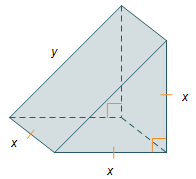# The volume of a cube is 1,000 cubic inches. What is the length of each side of the cube?

The volume of a cube is 1,000 cubic inches. What is the length of each side of the cube?

Answer 1

$\text{10 inch}$

#### Explanation:

Volume of cube is given by

${\text{Volume = (Edge Length)}}^{3}$

$\text{Edge length" = root(3)("Volume") = root(3)("1000 in"^3) = "10 in}$

#### Earn Coin

Coins can be redeemed for fabulous gifts.

Similar Homework Help Questions

• ### Length of of a side of a cube, if the volume is 1000 cubic meters

Length of of a side of a cube, if the volume is 1000 cubic meters?

• ### The volume of a cube is 125 cubic inches

The volume of a cube is 125 cubic inches. Find the surface area. I know that the volume formula is V=Bh where v=volume, B=area of the base, and h=height. How do I get the surface area though? Also, The surface area of a cube is 384 square cm. Find the volume. I think it's basically the same problem. So far, I know 384=Ph+2B, the surface area formula. I don't know how to find the variables though.The surface area of a...

• ### The volume of the triangular block is 4 cubic inches. What is the approximate length of...The volume of the triangular block is 4 cubic inches. What is the approximate length of y? Round to the nearest tenth of an inch. 1.4 in. 2.0 in. 2.8 in. 3.5 in. у х х х